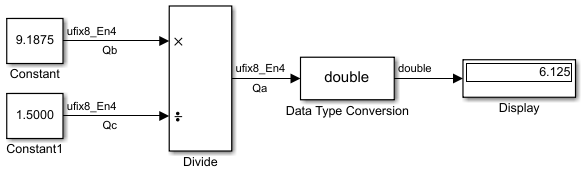## The Division Process

The C programming language provides access to integer division only for fixed-point data types. Depending on the size of the numerator, you can obtain some of the fractional bits by performing a shift prior to the integer division.

Suppose you want to divide two numbers. Each of these numbers is represented by an 8-bit word, and each has a binary-point-only scaling of 2-4. Additionally, the output is restricted to an 8-bit word with binary-point-only scaling of 2-4.

The division of 9.1875 by 1.5000 is shown in the following model.For this example,

`$\begin{array}{c}{Q}_{a}={2}^{-4-\left(-4\right)-\left(-4\right)}\left({Q}_{b}/{Q}_{c}\right)\\ ={2}^{4}\left({Q}_{b}/{Q}_{c}\right).\end{array}$`

Assuming a large data type was available, this could be implemented as

`${Q}_{a}=\frac{\left({2}^{4}{Q}_{b}\right)}{{Q}_{c}},$`

where the numerator uses the larger data type. If a larger data type was not available, integer division combined with four repeated subtractions would be used. Both approaches produce the same result, with the former being more efficient.

## SupportGet trial now